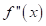# The significance of the sign f ' ( x ) over f ( x ) .### Single Variable Calculus: Concepts...

4th Edition
James Stewart
Publisher: Cengage Learning
ISBN: 9781337687805### Single Variable Calculus: Concepts...

4th Edition
James Stewart
Publisher: Cengage Learning
ISBN: 9781337687805

#### Solutions

Chapter 2, Problem 16RCC

(a)

To determine

## To find:The significance of the sign f'(x) over f(x) .

Expert Solution

The significance of the sign f'(x) over f(x) is When f'(x)>0 the function f(x) is increasing and for f'(x)<0 ,the function f(x) is decreases.

### Explanation of Solution

Given information:

The given statement is “The significance of the sign f'(x) over f(x) ”.

Calculation:

The derivative f'(x) represent the slope of the curve y=f(x) at the point (x,f(x)) .

When f'(x)>0 , the slope is positive and hence the function f(x) is increasing on that interval.

When f'(x)<0 , the slope is positive and hence the function f(x) is decreases on that interval.

Therefore, the significance of the sign f'(x) over f(x) is When f'(x)>0 the function f(x) is increasing and for f'(x)<0 ,the function f(x) is decreases.

(b)

To determine

### To find:The significance of the sign f''(x) over f(x) .

Expert Solution

The significance of the sign f''(x) over f(x) is When f''(x)>0 the function f(x) is concave upward and for f''(x)<0 ,the function f(x) is concave downward.

### Explanation of Solution

Given information:

The given statement is “The significance of the sign f''(x) over f(x) ”.

Calculation:

f''(x)=(f'(x))' , ifis positive, then f'(x) is an increasing function. Then the slope of the tangent lines of the curve y=f(x) increase from left to right.

If the value of x increases the slope of the curve becomes progressively larger and the curve bends upward. Such a curve called concave upward.

Therefore, if f''(x) is negative, then f'(x) is decreasing.

the slope of f(x) decreases from left to right and the curve bends downward. This curve is called concave downward.

From the above facts.

If f''(x)>0 on an interval, then f(x) is concave upward on that interval.

If f''(x)<0 on an interval, then f(x) is concave downward on that interval.

Therefore, the significance of the sign f''(x) over f(x) is When f''(x)>0 the function f(x) is concave upward and for f''(x)<0 ,the function f(x) is concave downward.

### Have a homework question?

Subscribe to bartleby learn! Ask subject matter experts 30 homework questions each month. Plus, you’ll have access to millions of step-by-step textbook answers!